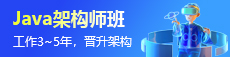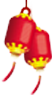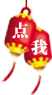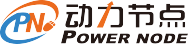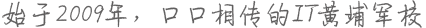Java基础学习分享之三元运算符

1，三元运算符的格式

(关系表达式) ? 表达式1 : 表达式2;

2，三元运算符的执行流程

int x = 10;
int y = 5;
int z;
z = (x > y) ? x : y;

3，获取两个数中的最大值

class Test1_Operator {
public static void main(String[] args) {
//获取三个整数中的最大值
int a = 10;
int b = 20;
int c = 30;
//先比较任意两个数的值,找出这两个数中的最大值
int temp = (a > b) ? a : b;
//用前两个数的最大值与第三个数比较,获取最大值
int max = (temp > c) ? temp : c;
System.out.println("max =" + max);
}
}

4，比较两个整数是否相同

class Test1_Operator {
public static void main(String[] args) {
//比较两个整数是否相同
int x = 10;
int y = 10;
boolean b = (x == y) ? true : false;
boolean b = (x == y);
System.out.println("b = " +  b);
}
}

Java基础视频教程下载